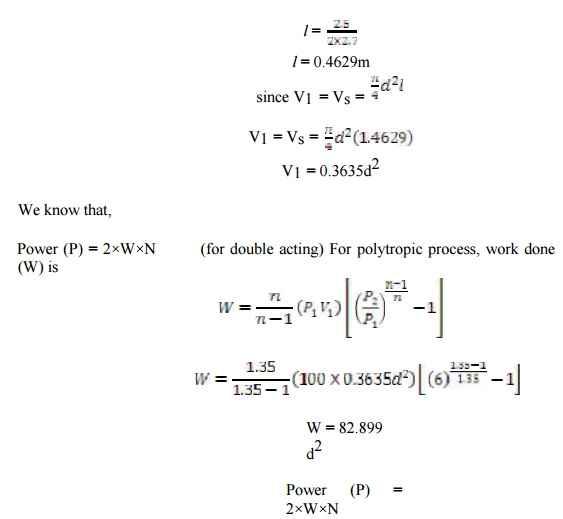Home | | Thermal Engineering I | Solved Problems: Air Compressors

# Solved Problems: Air Compressors

Mechanical and Electrical - Thermal Engineering - Air Compressors

SOLVED PROBLEMS

1.     A single stage double acting air compressor of 150KW power takes air in at 16 bar & delivers at 6 bar. The compression follows the law PV1.35 = C. the compressor runs at 160rpm with average piston speed of 150 m/min. Determine the size of the cylinder.

Given data

Power (P) = 150KW

Piston speed (2lN) = 150m /min

Speed (N) = 160rpm 160/6 0 = 2.7rps

Pressure (P1) = 1bar = 100 KN/m2

Pressure (P2) = 6bar = 600 KN/m2

PV1.35 = C,   n = 1.35

Hence it is a polytropic pr ocess.

To find

Size of the cylinder (d) ?

Solution

It is given that,

2lN = 2.5m/s150 = 2 × 82.899 d2 × 2.7

d2 = 0.3350

d = 0.57M

2.  A single stage single acting  reciprocating  air compressor is required  to  handle 30m3   of

free air per hour measured at 1 bar . the delivery pressure is 6.5 bar and the s peed is 450 r.p.m

allowing volumetric efficienc y of 75%;an isothermal   efficiency  of   76%  and mechanical

efficiency  of 80%  Find the indicated mean effective pressure and the po wer required  the

compressor

Given data

Volume                      V1 =30m2

Pressure                                P1=1 bar ,

P2=6.5 bar Speed               N=450 r.p.m

Volumetric efficiency  ηv=75%

Isothermal efficiency ηi=76%

Mechanical efficiency ηm=80%

To find

The indicated mean eff ective pressure

The power required to drive the compressor

Solution

Indicted Mean Effectiv e Pressure

We know that isothermal work doneTherefore the power required to drive the compressor = =2.56KW

Result

Indicated mean effective pressure pm=184.5KN/m2 The power required to drive the compressor =2.56KW

3. A two stages, single acting air compressor compresses air to 20bar. The air enters the L.P cylinder at 1bar and 27oc and leaves it at 4.7bar. The air enters the H.P. cylinder at 4.5bar and 27oc. the size of the L.P cylind er is 400mm diameter and 500mm stroke. The clearance volume In both cylinder is 4% of the respective stroke volume. The compressor runs at 200rpm, taking index of compression and ex pansion in the two cylinders as 1.3, estimate 1. The indicated power required to run the comp ressor; and 2. The heat rejected in the intercooler per minute.

Given data

Pressure (P4)= 20bar

Pressure (P1) = 1bar = 1× 105 N/m2

Temperature (T1) = 27oC = 27+273 = 300K Pressure (P2) = 4.7bar

Pressure (P3) = 4.5bar

Temperature (T3) = 27oC = 27+273 = 300K Diameter (D1) = 400mm 0.4m Stroke (L1) = 5 00mm = 0.5m

K = 0.04

N = 200rpm ; n = 1.3

To find

Indicated power required to run the compressor

Solution

We know the sw ept volume of the L.P cylinder= 2043.5× 103 J/min = 2034.5 KJ/min

Total indicated work done by the compressor,

W = WL + WH = 2123.3 + 2034 .5 = 4157.8 KJ/min

Indicated power required to run the compressor

= 4157.8 / 60

= 69.3KW

Study Material, Lecturing Notes, Assignment, Reference, Wiki description explanation, brief detail
Mechanical and Electrical : Thermal Engineering : Air Compressors : Solved Problems: Air Compressors |